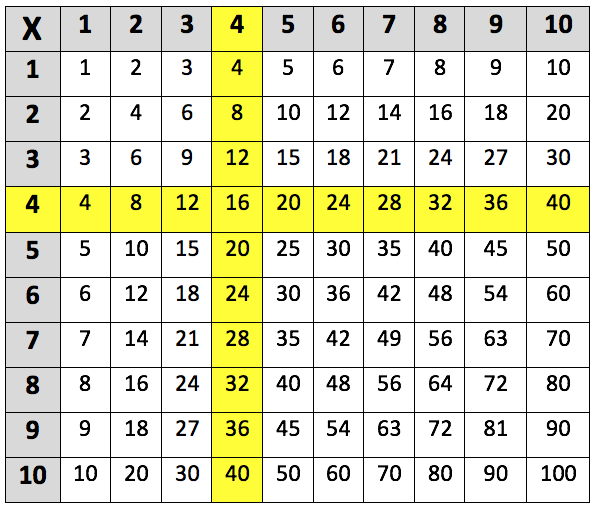Learning the 4 times table. The 4 times table up to 4 x 10 is highlighted in the multiplication grid below:This is the 4 times table up to 4 x 12:
4 x 1 = 4
4 x 2 = 8
4 x 3 = 12
4 x 4 = 16
4 x 5 = 20
4 x 6 = 24
4 x 7 = 28
4 x 8 = 32
4 x 9 = 36
4 x 10 = 40
4 x 11 = 44
4 x 12 = 48

## When do children learn the 4 times table?

In Year 2, children are taught the concept of multiplication and start by learning the 2, 5 and 10 times tables.

In Year 3, teachers move on to the 3, 4 and 8 times tables. Children should also be able to use practical and written methods of multiplying and dividing two-digit numbers. For example:

12 x 4 = 48

By Years 5 and 6, children are expected to be confident in their times tables up to 12 and should be able to use their knowledge of times tables to help them with other aspects of maths, including percentages, fractions and long multiplications.

Children should also be learning the division facts for the 4 times table, such as:

4 ÷ 4 = 1, 8 ÷ 4 = 2 and 12 ÷ 4 = 3

## How to help children with the 4 times table?

As with other times tables, there are a few tricks and patterns in the 4 times table which can make learning them easier. Firstly, every number which is a multiple of 4 is an even number, and so will always end in 0, 2, 4, 6 or 8. This should help children to easily recognise whether a number is a multiple of 4 or not.

It might also help children if they know that multiplying a number by 4 is the same as doubling the number twice. For example:

8 x 4 is the same as 8 x 2 x 2

8 x 2 = 16, then 16 x 2 = 32. So 8 x 4 = 32

Breaking down the multiplication like above will be beneficial for children who are confident with their 2 times tables and with doubling numbers.

One way of helping children learn their times tables if by making it an interactive process. This can be done by using building blocks. For the 4 times table, create a stack of 4 blocks, 8 blocks, 12 blocks etc. Then ask your child to point at or hold each stick of numbers as they count up in 4s. Once they become more confident with this, you could then ask your child a question, such as 4 x 3, and get them to hold up the stick of numbers which represents the correct answer.

## How does Learning Street help children with the 4 times table?

Repetition is key to learning your 4 times table, and in the Learning Street 8 year old courses, there is a constant focus on always practising times tables as this is the best way to improve. This is through times tables tests (to improve accuracy), tables races (to improve time), mental mathematics, maths problem solving and other fun activities such as mystery pictures.

Without a good knowledge of times tables, it can lead to problems in SATs, GCSEs and A Levels.

## Our Courses

Click through to review the courses we have available

##### Get Started

Learning Street structured courses make home study easier and more successful. There's no need to wonder which books to buy or what to do next. Everything is planned for you.

• Proven to deliver excellent results
• Used by families, private tutors and schools
• Fully planned and structured